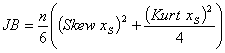# Jarque-Bera test

The Jarque-Bera test is used to check hypothesis about the fact that a given sample x is a sample of normal random variable with unknown mean and dispersion. As a rule, this test is applied before using methods of parametric statistics which require distribution normality.

# Criterion

This test is based on the fact that skewness and kurtosis of normal distribution equal zero. Therefore, the absolute value of these parameters could be a measure of deviation of the distribution from normal. Using the sample Jarque-Bera statistic is calculated:(here n is a size of sample), then p-value is computed using a table of distribution quantiles. It should be noted that as n increases, JB-statistic converges to chi-square distribution with two degrees of freedom, so sometimes in practice table of chi-square distribution quantiles is used. However, this is a mistake - convergence is too slow and irregular.

For example, even if n = 70 (which is rather big value) and having JB = 5 chi-square distribution quantiles gives us p-value p = 0.08, whereas real p-value equals 0.045. So, we can accept the wrong hypothesis. Therefore it's better to use the specially created table of Jarque-Bera distribution quantiles.

# Distribution quantiles

To create this table, the Monte-Carlo method was used. The program in C++ had generated 3600000 samples of n normal numbers (at that, a high-quality random number generator was used). Having these samples 3600000 values JB(x) were calculated. These values were used to construct tables of quantiles for given n. This was done for each n from {5, 6, 7, ..., 198, 199, 200, 201, 251, 301, 351, ..., 1901, 1951} . Total calculation time was several tens of machine hours.

The table created was too big (2.5 Mb in binary format), so the following step was to compress it: JB(x) distribution for the key n was saved using piecewise-polynomial approximation, intermediate values are found using interpolation. For n > 1401 asymptotic approximation is used.

# Quality of the table

We think that the approximation table is good enough for practice needs. You can find relative errors for different p-values in the following table:

```p-value		   relative error (5≤N≤1951)
[1, 0.1]            < 1%
[0.1, 0.01]         < 2%
[0.01, 0.001]       < 6%
[0.001, 0]          wasn't measured
```

We should note that the most accurate p-values belong to interval [0, 0.01]. This interval is used to make a decision most often. Accuracy decreasing in [0.01, 0.001] is determined by the fact that the less p-value, the less probability to get it if a null hypothesis is accepted, and the more tests are required to find the corresponding distribution quantile.

To calculate p-values in interval [0.001, 0] asymptotic approximation is used. The author believes that this method allows us to get credible results in a reasonable interval. The quality of such approximation wasn't measured because of the considerable machine time required to perform such measurement.

# Download ALGLIB for C++ / C# / ...

ALGLIB Project offers you two editions of ALGLIB:

ALGLIB Free Edition:delivered for freeoffers full set of numerical functionalityextensive algorithmic optimizationsno low level optimizationsnon-commercial license

ALGLIB Commercial Edition:flexible pricingoffers full set of numerical functionalityextensive algorithmic optimizationshigh performance (SMP, SIMD)commercial license with support plan

## ALGLIB 3.15.0 for C++C++ library.
Delivered with sources.
Monolithic design.
Extreme portability.
Editions:   FREE   COMMERCIAL

## ALGLIB 3.15.0 for C#C# library with native kernels.
Delivered with sources.
VB.NET and IronPython wrappers.
Extreme portability.
Editions:   FREE   COMMERCIAL

## ALGLIB 3.15.0 for DelphiDelphi wrapper around C core.
Delivered as precompiled binary.
Compatible with FreePascal.
Editions:   FREE   COMMERCIAL

## ALGLIB 3.15.0 for CPythonCPython wrapper around C core.
Delivered as precompiled binary.
Editions:   FREE   COMMERCIAL